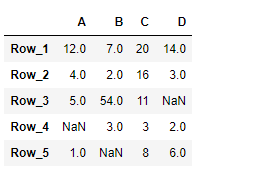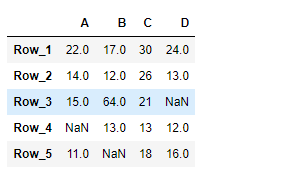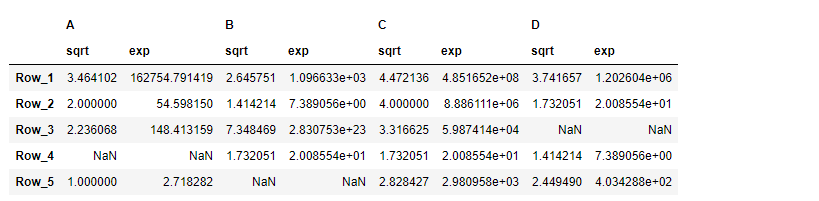# Python | Pandas DataFrame.transform

Pandas DataFrame is a two-dimensional size-mutable, potentially heterogeneous tabular data structure with labeled axes (rows and columns). Arithmetic operations align on both row and column labels. It can be thought of as a dict-like container for Series objects. This is the primary data structure of the Pandas.

Pandas` DataFrame.transform()` function call func on self producing a DataFrame with transformed values and that has the same axis length as self.

Syntax: DataFrame.transform(func, axis=0, *args, **kwargs)

Parameter :
func : Function to use for transforming the data
axis : {0 or ‘index’, 1 or ‘columns’}, default 0
*args : Positional arguments to pass to func.
**kwargs : Keyword arguments to pass to func.

Returns : DataFrame

Example #1 : Use `DataFrame.transform()` function to add 10 to each element in the dataframe.

 `# importing pandas as pd ` `import` `pandas as pd ` ` `  `# Creating the DataFrame ` `df ``=` `pd.DataFrame({``"A"``:[``12``, ``4``, ``5``, ``None``, ``1``],  ` `                   ``"B"``:[``7``, ``2``, ``54``, ``3``, ``None``],  ` `                   ``"C"``:[``20``, ``16``, ``11``, ``3``, ``8``],  ` `                   ``"D"``:[``14``, ``3``, ``None``, ``2``, ``6``]})  ` ` `  `# Create the index ` `index_ ``=` `[``'Row_1'``, ``'Row_2'``, ``'Row_3'``, ``'Row_4'``, ``'Row_5'``] ` ` `  `# Set the index ` `df.index ``=` `index_ ` ` `  `# Print the DataFrame ` `print``(df) `

Output :Now we will use `DataFrame.transform()` function to add 10 to each element of the dataframe.

 `# add 10 to each element of the dataframe ` `result ``=` `df.transform(func ``=` `lambda` `x : x ``+` `10``) ` ` `  `# Print the result ` `print``(result) `

Output :As we can see in the output, the `DataFrame.transform()` function has successfully added 10 to each element of the given Dataframe.

Example #2 : Use `DataFrame.transform()` function to find the square root and the result of euler’s number raised to each element of the dataframe.

 `# importing pandas as pd ` `import` `pandas as pd ` ` `  `# Creating the DataFrame ` `df ``=` `pd.DataFrame({``"A"``:[``12``, ``4``, ``5``, ``None``, ``1``],  ` `                   ``"B"``:[``7``, ``2``, ``54``, ``3``, ``None``],  ` `                   ``"C"``:[``20``, ``16``, ``11``, ``3``, ``8``],  ` `                   ``"D"``:[``14``, ``3``, ``None``, ``2``, ``6``]})  ` ` `  `# Create the index ` `index_ ``=` `[``'Row_1'``, ``'Row_2'``, ``'Row_3'``, ``'Row_4'``, ``'Row_5'``] ` ` `  `# Set the index ` `df.index ``=` `index_ ` ` `  `# Print the DataFrame ` `print``(df) `

Output :Now we will use `DataFrame.transform()` function to find the square root and the result of euler’s number raised to each element of the dataframe.

 `# pass a list of functions ` `result ``=` `df.transform(func ``=` `[``'sqrt'``, ``'exp'``]) ` ` `  `# Print the result ` `print``(result) `

Output :As we can see in the output, the `DataFrame.transform()` function has successfully performed the desired operation on the given dataframe.

My Personal Notes arrow_drop_upCheck out this Author's contributed articles.

If you like GeeksforGeeks and would like to contribute, you can also write an article using contribute.geeksforgeeks.org or mail your article to contribute@geeksforgeeks.org. See your article appearing on the GeeksforGeeks main page and help other Geeks.

Please Improve this article if you find anything incorrect by clicking on the "Improve Article" button below.

Article Tags :

Be the First to upvote.

Please write to us at contribute@geeksforgeeks.org to report any issue with the above content.